Скачать презентацию REVIEW CS 1033 Pixel and Resolution http www

• Количество слайдов: 10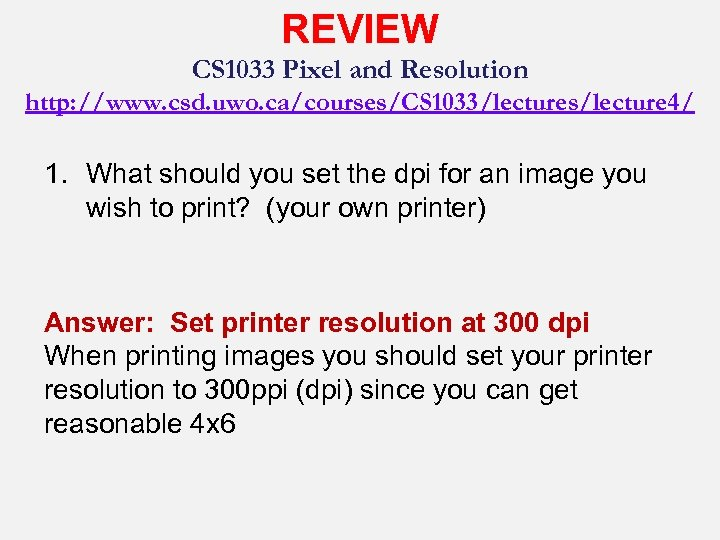REVIEW CS 1033 Pixel and Resolution http: //www. csd. uwo. ca/courses/CS 1033/lectures/lecture 4/ 1. What should you set the dpi for an image you wish to print? (your own printer) Answer: Set printer resolution at 300 dpi When printing images you should set your printer resolution to 300 ppi (dpi) since you can get reasonable 4 x 6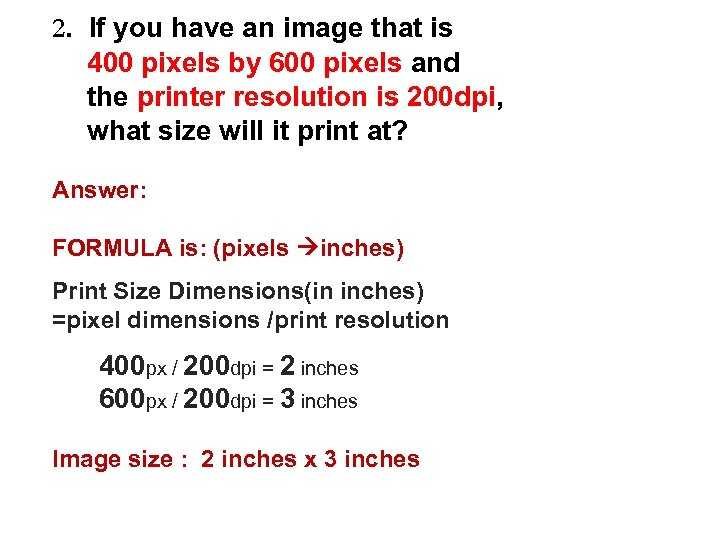2. If you have an image that is 400 pixels by 600 pixels and the printer resolution is 200 dpi, what size will it print at? Answer: FORMULA is: (pixels inches) Print Size Dimensions(in inches) =pixel dimensions /print resolution 400 px / 200 dpi = 2 inches 600 px / 200 dpi = 3 inches Image size : 2 inches x 3 inches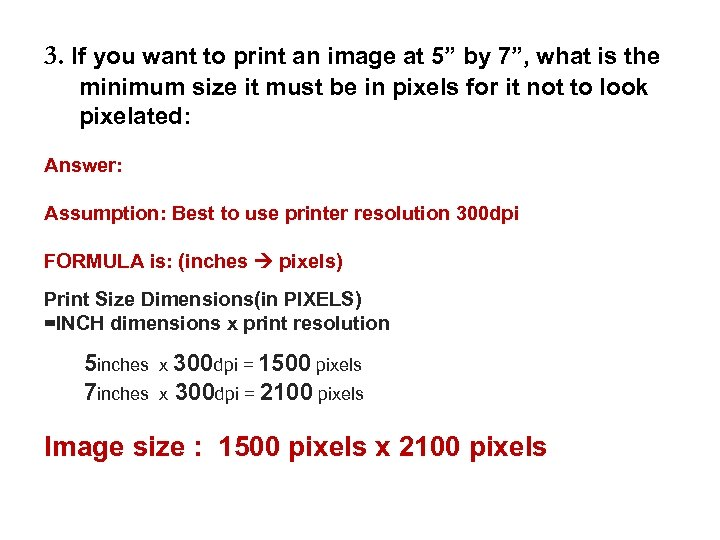3. If you want to print an image at 5” by 7”, what is the minimum size it must be in pixels for it not to look pixelated: Answer: Assumption: Best to use printer resolution 300 dpi FORMULA is: (inches pixels) Print Size Dimensions(in PIXELS) =INCH dimensions x print resolution 5 inches x 300 dpi = 1500 pixels 7 inches x 300 dpi = 2100 pixels Image size : 1500 pixels x 2100 pixels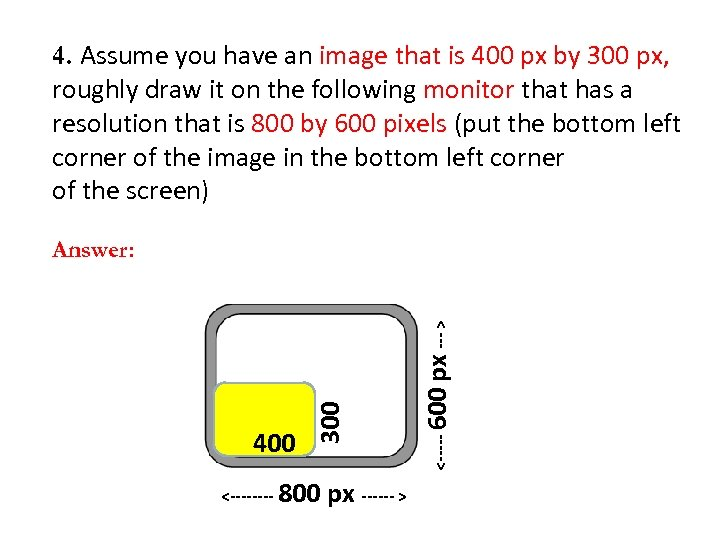4. Assume you have an image that is 400 px by 300 px, roughly draw it on the following monitor that has a resolution that is 800 by 600 pixels (put the bottom left corner of the image in the bottom left corner of the screen) <---- 800 px ------ > <----- 600 400 300 px --- > Answer: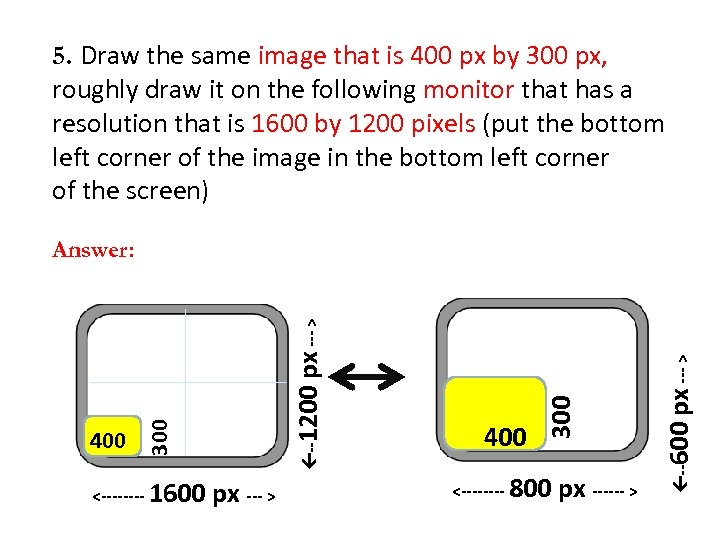5. Draw the same image that is 400 px by 300 px, roughly draw it on the following monitor that has a resolution that is 1600 by 1200 pixels (put the bottom left corner of the image in the bottom left corner of the screen) px --- > <---- 800 px ------ > px --- > --600 <---- 1600 400 300 400 --1200 px --- > Answer: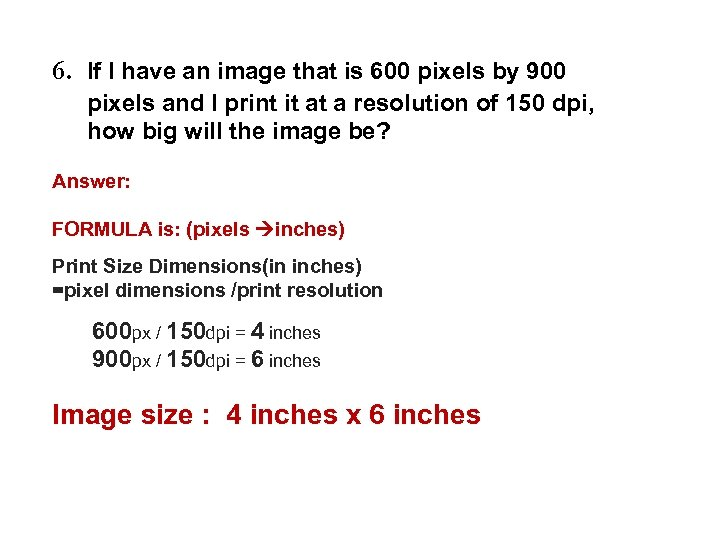6. If I have an image that is 600 pixels by 900 pixels and I print it at a resolution of 150 dpi, how big will the image be? Answer: FORMULA is: (pixels inches) Print Size Dimensions(in inches) =pixel dimensions /print resolution 600 px / 150 dpi = 4 inches 900 px / 150 dpi = 6 inches Image size : 4 inches x 6 inches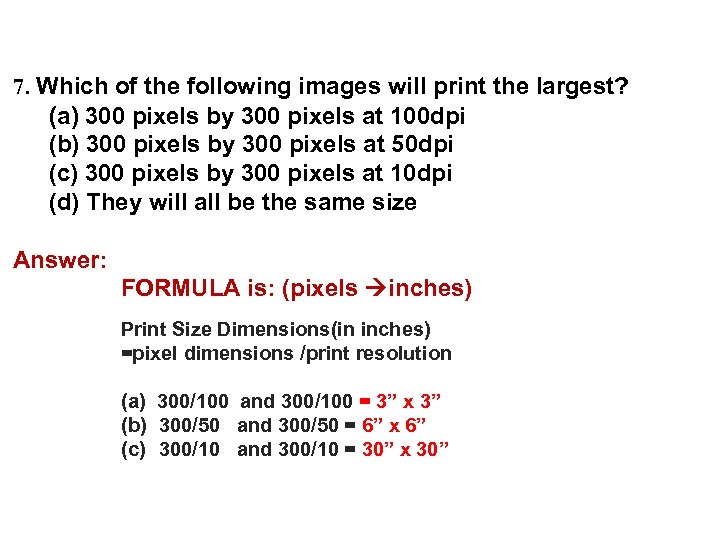7. Which of the following images will print the largest? (a) 300 pixels by 300 pixels at 100 dpi (b) 300 pixels by 300 pixels at 50 dpi (c) 300 pixels by 300 pixels at 10 dpi (d) They will all be the same size Answer: FORMULA is: (pixels inches) Print Size Dimensions(in inches) =pixel dimensions /print resolution (a) 300/100 and 300/100 = 3” x 3” (b) 300/50 and 300/50 = 6” x 6” (c) 300/10 and 300/10 = 30” x 30”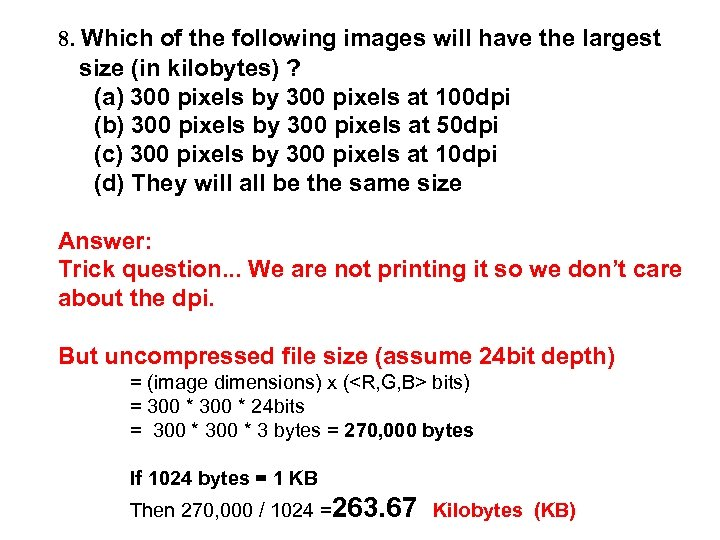8. Which of the following images will have the largest size (in kilobytes) ? (a) 300 pixels by 300 pixels at 100 dpi (b) 300 pixels by 300 pixels at 50 dpi (c) 300 pixels by 300 pixels at 10 dpi (d) They will all be the same size Answer: Trick question. . . We are not printing it so we don’t care about the dpi. But uncompressed file size (assume 24 bit depth) = (image dimensions) x ( bits) = 300 * 24 bits = 300 * 3 bytes = 270, 000 bytes If 1024 bytes = 1 KB Then 270, 000 / 1024 =263. 67 Kilobytes (KB)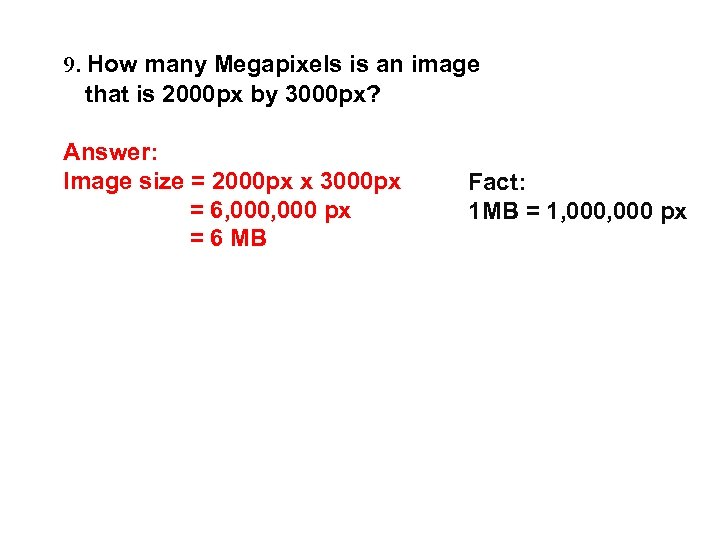9. How many Megapixels is an image that is 2000 px by 3000 px? Answer: Image size = 2000 px x 3000 px = 6, 000 px = 6 MB Fact: 1 MB = 1, 000 px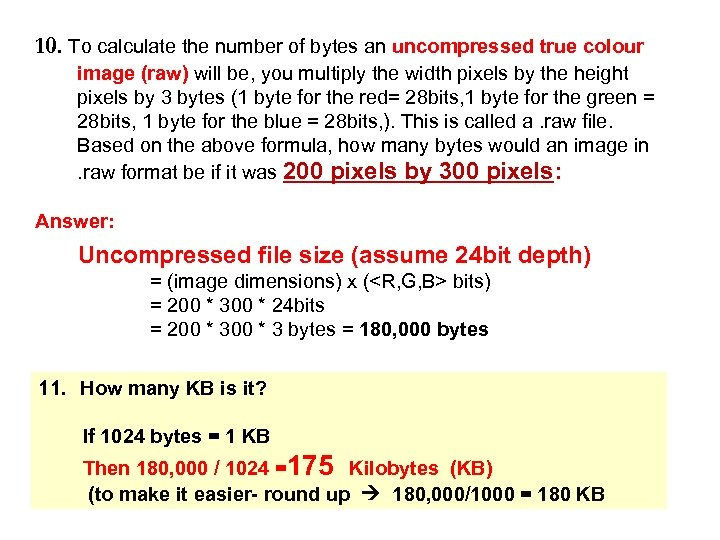10. To calculate the number of bytes an uncompressed true colour image (raw) will be, you multiply the width pixels by the height pixels by 3 bytes (1 byte for the red= 28 bits, 1 byte for the green = 28 bits, 1 byte for the blue = 28 bits, ). This is called a. raw file. Based on the above formula, how many bytes would an image in. raw format be if it was 200 pixels by 300 pixels: Answer: Uncompressed file size (assume 24 bit depth) = (image dimensions) x ( bits) = 200 * 300 * 24 bits = 200 * 3 bytes = 180, 000 bytes 11. How many KB is it? If 1024 bytes = 1 KB Then 180, 000 / 1024 =175 Kilobytes (KB) (to make it easier- round up 180, 000/1000 = 180 KB